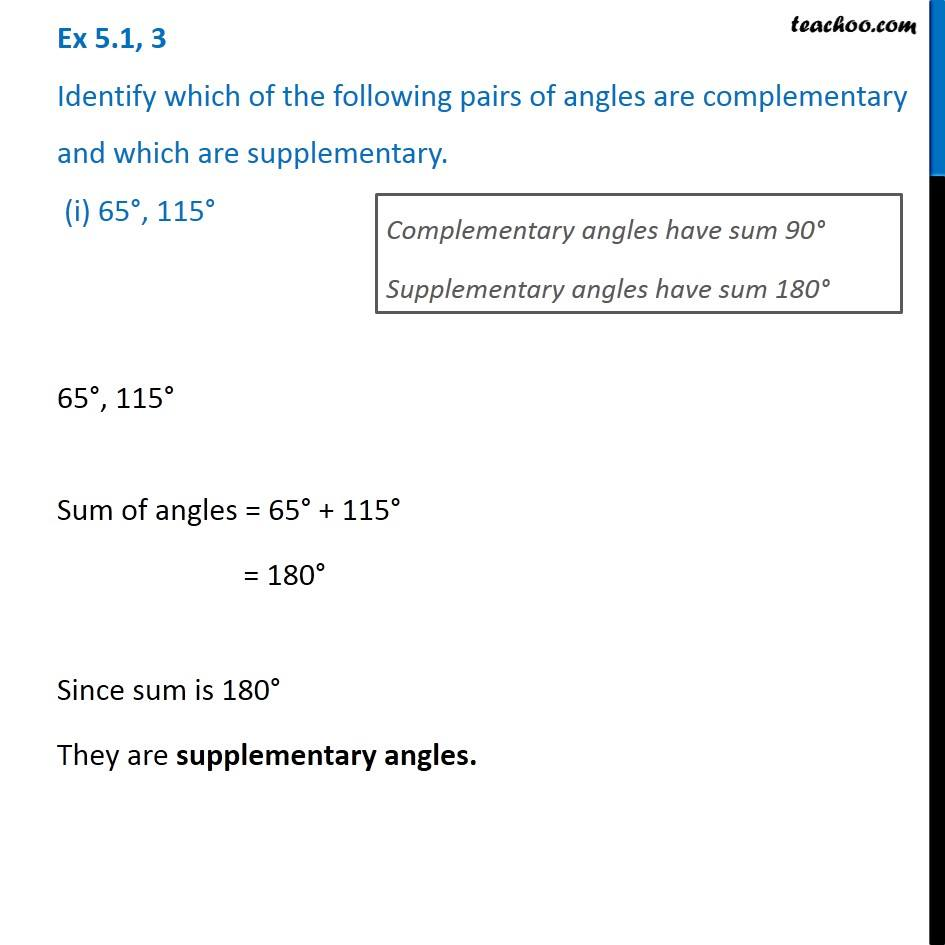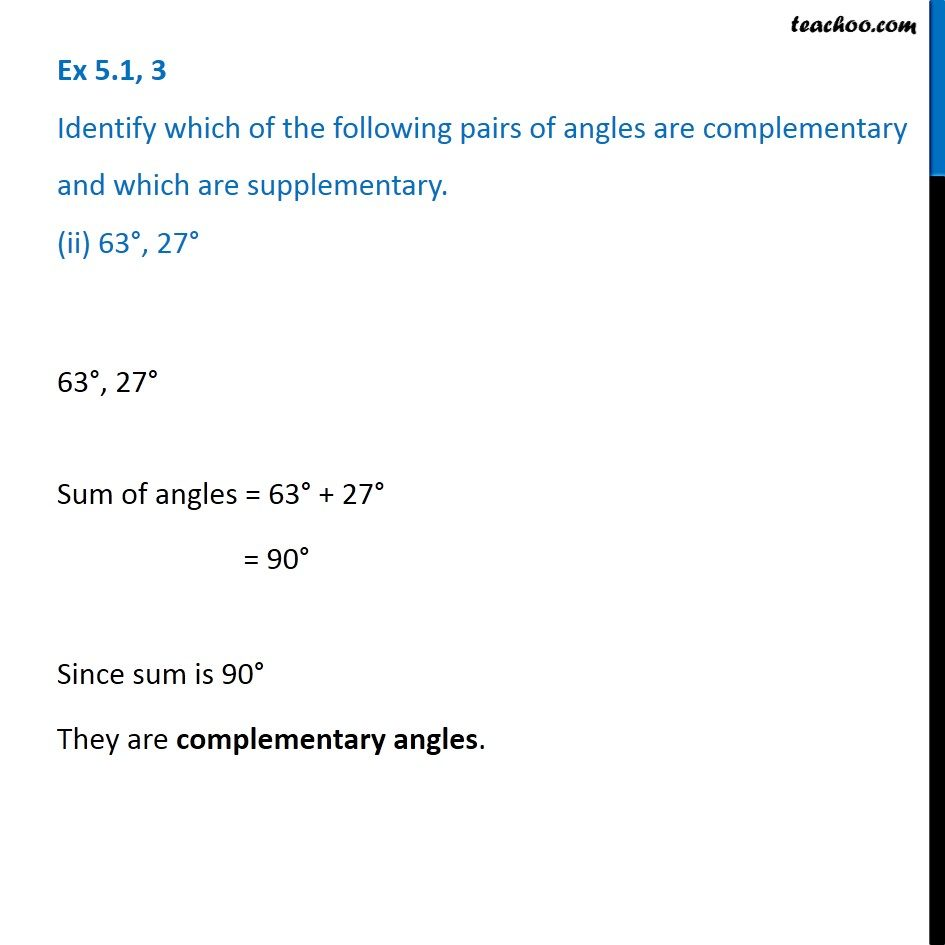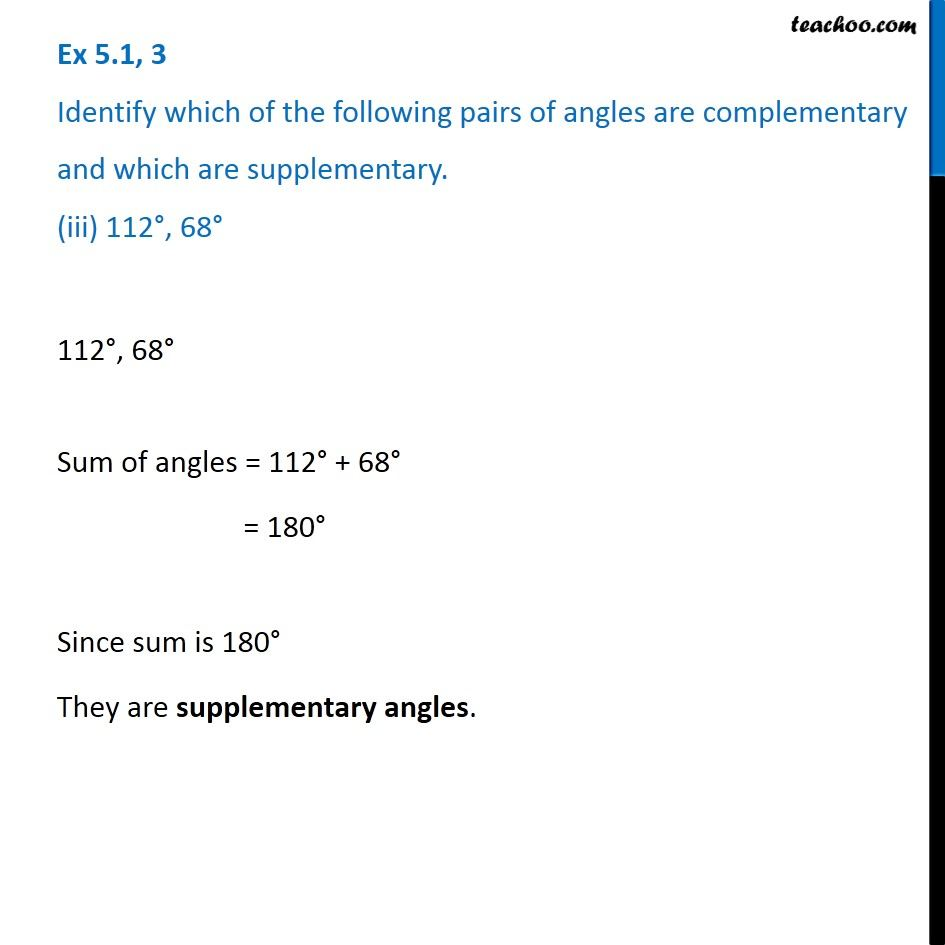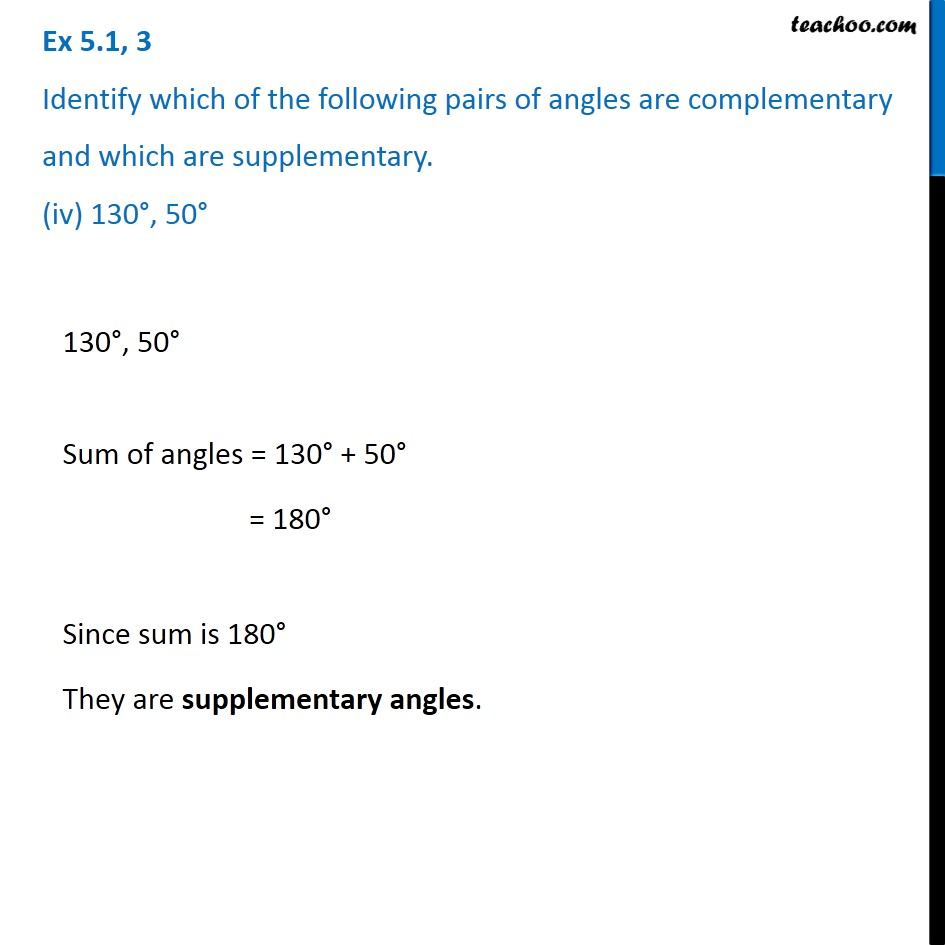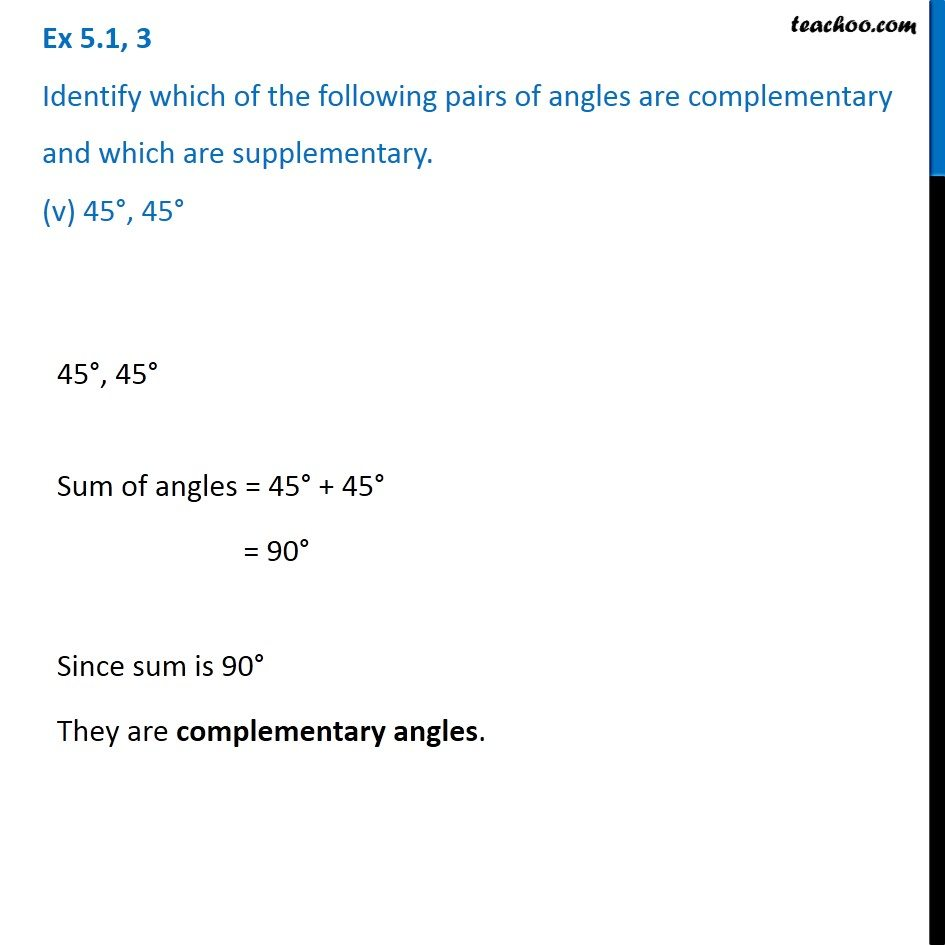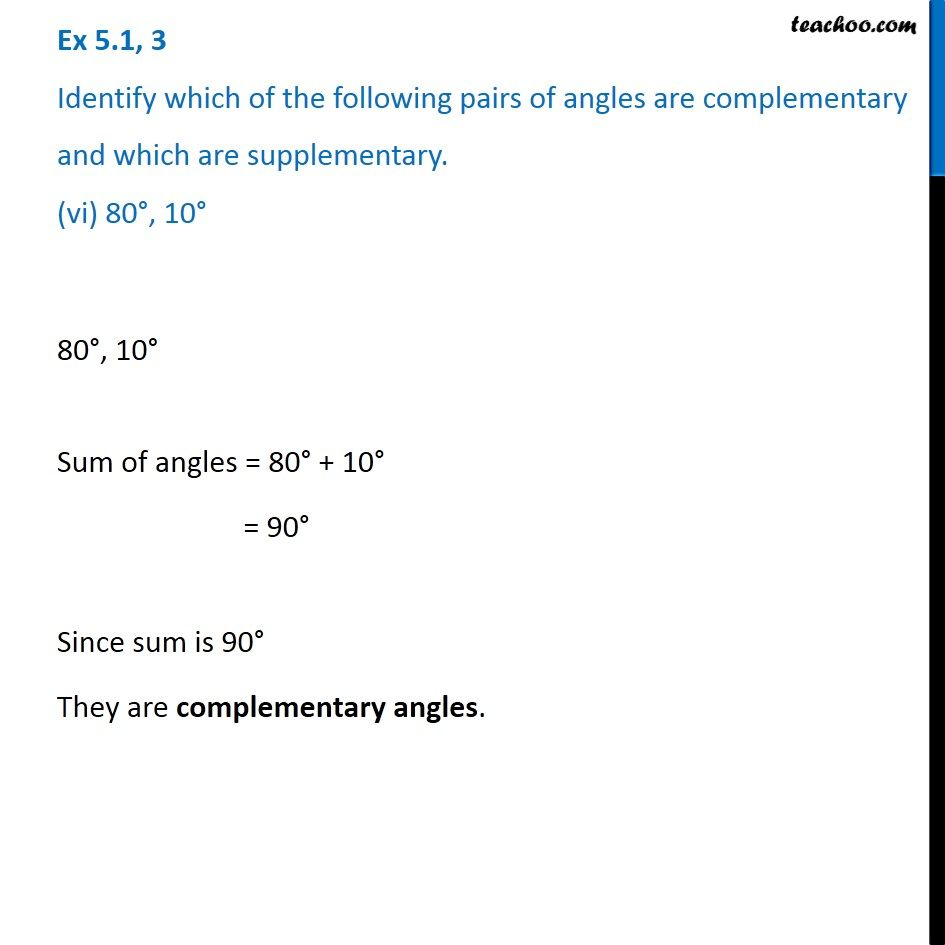Subscribe to our Youtube Channel - https://you.tube/teachoo

1. Chapter 5 Class 7 Lines and Angles
2. Serial order wise
3. Ex 5.1

Transcript

Ex 5.1, 3 Identify which of the following pairs of angles are complementary and which are supplementary. (i) 65°, 115°Complementary angles have sum 90° Supplementary angles have sum 180° 65°, 115° Sum of angles = 65° + 115° = 180° Since sum is 180° They are supplementary angles. Ex 5.1, 3 Identify which of the following pairs of angles are complementary and which are supplementary. (ii) 63°, 27° 63°, 27° Sum of angles = 63° + 27° = 90° Since sum is 90° They are complementary angles. Ex 5.1, 3 Identify which of the following pairs of angles are complementary and which are supplementary. (iii) 112°, 68° 112°, 68° Sum of angles = 112° + 68° = 180° Since sum is 180° They are supplementary angles. Ex 5.1, 3 Identify which of the following pairs of angles are complementary and which are supplementary. (iv) 130°, 50° 130°, 50° Sum of angles = 130° + 50° = 180° Since sum is 180° They are supplementary angles. 130°, 50° Sum of angles = 130° + 50° = 180° Since sum is 180° They are supplementary angles. 45°, 45° Sum of angles = 45° + 45° = 90° Since sum is 90° They are complementary angles. Ex 5.1, 3 Identify which of the following pairs of angles are complementary and which are supplementary. (vi) 80°, 10°80°, 10° Sum of angles = 80° + 10° = 90° Since sum is 90° They are complementary angles.

Ex 5.1